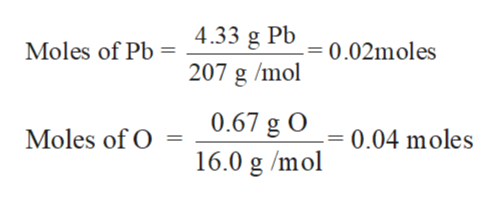# A 5.00 gram sample of an oxide of lead PbxOy contains 4.33 g of lead. Determine the simplest formula for the compound.

Question
35 views

A 5.00 gram sample of an oxide of lead PbxOy contains 4.33 g of lead. Determine the simplest formula for the compound.

check_circle

Step 1

The empirical formula is the chemical formula of any compound that represents the proportions of each elements that is present in the given compound. It does not show the actual number of element as well as cannot give any information about arrangement of atoms in the formula of compound.

Step 2

Mass of sample = 5.00 g

Mass of Pb = 4.33 g

Hence mass of O = 5.00 – 4.33 = 0.67 g

Step 3

Calculate the moles of Pb and O:

Molar mass of Pb = 20...help_outlineImage TranscriptioncloseMoles of Pb 4.33 g Po= 0.02moles 207 g /mol 0.67 g O 0.04 moles Moles of O 16.0 g /mol fullscreen

### Want to see the full answer?

See Solution

#### Want to see this answer and more?

Solutions are written by subject experts who are available 24/7. Questions are typically answered within 1 hour.*

See Solution
*Response times may vary by subject and question.
Tagged in

### Other